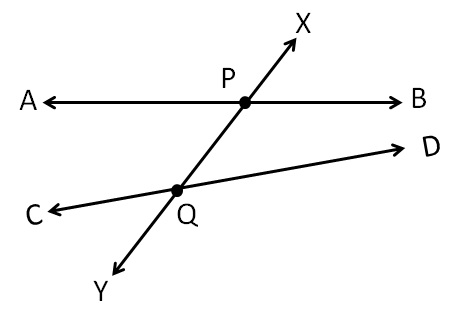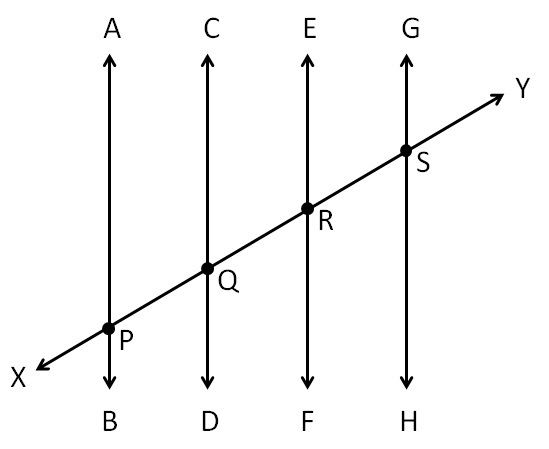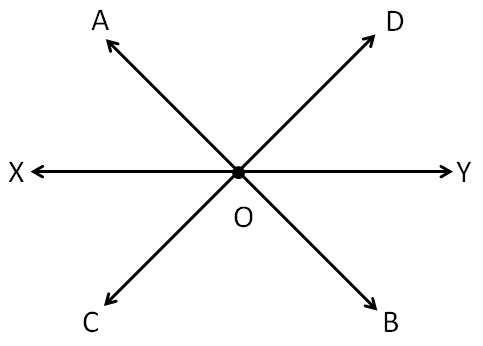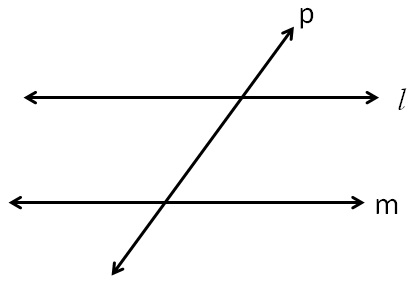Angles made by the transversal - Theory

Chapter 5 Class 7 Lines and Angles
Concept wise

Transversal is a line which intersects two or more lines at distinct pointsSince line XY intersects line AB & CD, at distinct points P & Q

It is transversal.

It can also intersect more than 2 linesHere XY intersects these 4 lines.

At distinct points P, Q, R, S.

So, it is a transversalHere, line XY intersects AB & CD

at point O.

Since it does not intersect at distinct points,

it is not a transversal.

Generally,

We talk about transversal of parallel linesHere,

line l and m are parallel

and line p is the transversal of line l & m.

Let’s learn the angles formed by the transversal.

Learn in your speed, with individual attention - Teachoo Maths 1-on-1 Class